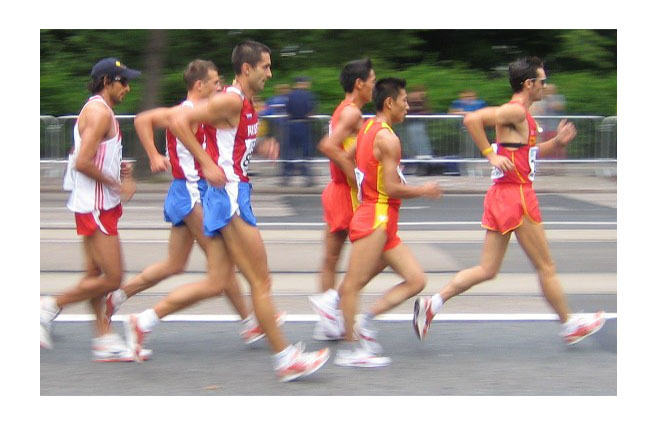4.12

## UNSW SydneyRacewalkers in Helsinki, Finland

# The power of exercise

It is commonly accepted that regular exercise adds years onto life, but what exactly is the power of exercise? And is this power a power law?

In this step, we will

• look at real life data and use it to derive a simple power law

• get more practice using a log-log plot

• learn that we ought to exercise more.

## Is exercise really good for you?

Researchers from the Harvard-affiliated Brigham and Women’s Hospital published an article in 2012, which investigated the correlation between regular exercise and rates of mortality. In that paper we find the rather suggestive data plot showing years of increased life expectancy (measured in years) versus Leisure time physical activity (measured in Metabolic Equivalent Task Hours/Week)This bears a good resemblance to the power laws we’ve been investigating.

Q1 (E): What kind of power law do you think this could be? What do you guess the exponent is?

Here are the rough values for these data points

 Physical activity $\normalsize A$ (hours/week) Years $\normalsize Y$ of life gained 2.5 1.8 4.5 2.6 9.5 3.4 20 4.2 27 4.6

And here are the rough values for the logarithms of the data points

 $\normalsize \ln(A)$ $\normalsize \ln(Y)$ 0.92 0.59 1.50 0.96 2.25 1.22 3.00 1.44 3.30 1.53

If we plot this data on a log-log plot we get the followingThe relationship between these points looks fairly linear.

Q2 (M): What line do you think best fits this data? What does this means for the power law? Put your answer in the discussion section.

## A line of best fit

Eye-balling the graph we estimate a slope of about $\normalsize \frac{1}{3}$. The intercept with the $\normalsize \ln(Y)$-axis looks to be about $\normalsize 0.3$. This gives an equation

For an answer that involves less guess work, an advanced student could calculate that the least squares line of best fit is

Try asking Wolfram Alpha to verify this.

## Getting a power law

The linear relation between logarithms yields a power law relation between the original variables. When we exponentiate our line $(\ref{1})$, we get the equation

or just

This is a power law.## Even a little exercise is good for you

So life expectancy scales to the $\normalsize 0.38$ power of hours of weekly exercise. One way of reading this is that the benefit of exercise builds up rather quickly, so that even a small amount of exercise per week has a significant benefit. Another way to read this is that exercising gives diminishing returns, so that your first three hours of exercise is worth more than your next three hours etc.

Q2 (E): How much longer are you going to live if you exercise $\normalsize 2,6,10,14$ and $\normalsize 18$ hours per week?

Q3 (M): If Chad exercises twice as much as Brad, and Brad is expecting $\normalsize 3$ more years of life, how much more life expectancy should Chad expect?

Q4 (C): One could argue that the additional years of life are not the primary bonus of getting in better shape – that the quality of life is likely also improved. Our next Future Learn course therefore clearly out to be Exercise for Humans! What do you think about this topic – are there some other important points to be made??

A1. A rough guess would be a power law with exponent $\normalsize k=\frac{1}{2}$.

A2. Here is how many additional years that physical activity will add to your life:

hours exercised per week additional years of life
2 1.8
6 2.7
10 3.3
14 3.8
18 4.1

A3. If we double the hours of exercise per week then the expected additional years of life should multiply by $2^{0.38}\approx 1.3$. So Chad should expect to have $\normalsize 3 \times 1.3 = 3.9$ years of additional life. Way to go Chad.

A4. See discussion.

Harvard Gazette: How much exercise is enough? Nov 6 2012

Leisure Time Physical Activity of Moderate to Vigorous Intensity and Mortality: A Large Pooled Cohort Analysis